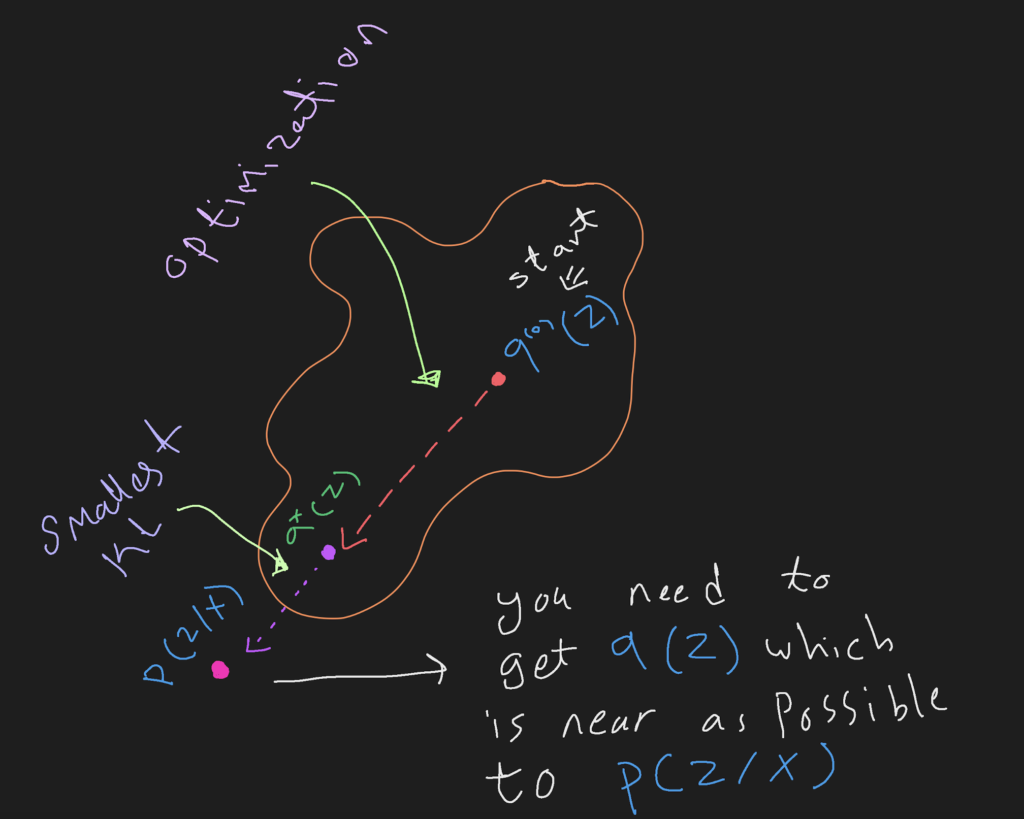# What is Evidence Lower Bound (ELBO)?

The evidence lower bound (ELBO) is an important quantity that lies at the core of a number of important algorithms in probabilistic inference such as expectation-maximization and variational inference. To understand these algorithms, it is helpful to understand the ELBO.

• Variational inference and KL divergence
• Evidence lower bound derivation

### Variational inference

Variational inference main goal is to find a relationship between Z and X, where Z is a latent variable and X are our observations {X1,X2, X3, ….. XN}. for this, Bayes’ Rule is used and we always interested in the posterior distribution p(Z|X), however posterior distribution is intractable (can’t be calculated), because we can’t compute evidence p(X). So, the main goal of variational inference is to find a simple tractable distribution q(Z), which is close as possible to p(Z|X). Kullback-Leibler (KL) divergence is defined to measure the similarity between two distribution (see Eq. 1)

where q(Z) is from a family of distributions Q. and DKL is divergence between the predicted q(Z) distribution and the posterior distribution p(Z/X).Figure 1. Iteratively minimizing the KL divergence between the approximating at iteration t q(t) and the desired posterior P(Z/X)

Variational inference and ELBO equation is used in many application and models, e.g. Variational Autoencoders  however this post will be focused on the derivation of the ELBO and how we can reach a formula that made it easy to optimize the q(Z) distribution.

### Evidence Lower Bound Derivation

In this subsection we will go step by step of how we get the right formula to approximate q(Z|X) distribution using ELBO, as, finding posterior p(Z|X) is impossible because of the intractable evidence p(x) (see Figure 2).

Kl divergence  is a formula to approximate and quantify difference between two distribution, and in our case we want the distance between p(Z|X) and the approximated q(Z|X) is small as possible (see Figure 3).

As we see in (Figure 4) we first, replaced the log by subtracting both quantities. then, we can see that the posterior distribution p(Z|X) can’t be computed (Figure 3), so, instead we will replace it with the joint probability over the prior p(Z, X) / p(X). (see Figure 4).

As, The posterior p(Z|X) is what we are trying to approximate. We perform this approximation using the distribution q, whose parameters we plan to determine through an optimization process, and from which we are aware of how to sample. As a result, even though the distribution q differs from that of p, it would still be close to or for z|x. In other words, it is a distribution that is “assumed” for “z|x”.

Indicated by the symbol or notation “E q,” this expectation has a probability distribution of “q.” The probability distribution is implied by whatever appears in the subscript of the symbol E. and since q is a distribution of z given x during the entire lesson (i.e., Z|X), the notations E q and E q(Z|X) are identical, i.e., q and q(Z|X) are identical. Because of this, instead of q when it will be expanded, it will be q(Z|X) q(X) and not only q(x). (see Eq. 2)

And from this fact we can expand E q [p(X)] and continue our derivation as shown in (Figure 5)

Now we can put the log likelihood of our observations p(X) on the left side of the equation, and by maximizing component 1 in (Figure 6), the KL divergence (component 2) as a result will be minimized directly which is our main goal to make the approximated distribution similar as possible to the actual distribution.

if you don’t understand why if component 1 increases then component 2 (KL divergence) will decrease directly, then let’s say as an example, if we have an equation as follow, fixed amount = a+b, then if `a` increases, `b` must decrease in order to respect above equation.

The final simplification of (component 1) starts with extended the joint probability p(z,x) into two parts then the equation will contain two parts, the first one the called the reconstraction part which repressents The Mean sqaured error between the input data and the reconstracted output and the second part is the KL divergence between the approximated distribution q(Z/X) and the prior p(X) see (Figure 7).

•